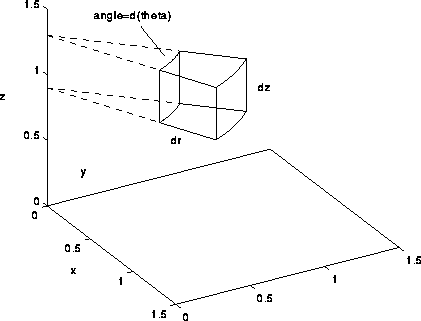# Acceleration due to gravity at the centre of a hemisphere

## Homework Statement

find the acceleration due to gravity at the centre of a solid hemisphere.

## Homework Equations

##F=\frac{GMm}{r^2}##

## The Attempt at a Solution

i decided to go for cylindrical coordinayes (which is way beyond my syllabus). I did some research though.
let me take a point P(r,θ,z) inside the sphere amd an elemental volume dV at P. This P exerts a force dF. But there is also a point Q(r,θ+180°,z) which cancells out the horizontal component of dF. let the line OP make an angle φ with the Z axis. (assuming the hemisphere lies on the xy plane with centre at O)
##dg=\frac{Gdm}{r^2+z^2}cos\phi##
##dg=\frac{G\rho dV}{{(r^2+z^2)}^{3/2}}##
now dV=dz.dr.rdθ
Is this expresion for dV true for all cases? how do you get that expression for dV?
(i found that expression on some video).

now i have to integrate the expression.
so $$g=\int_{\theta=0}^{2\pi}\int_{r=0}^R\int_{z=0}^?f(r,\theta,z)dz.dr.rd\theta$$
im finding it difficult to find upper limit for z.

Last edited:

Why not use spherical coordinates $(r,\theta,\varphi)$ instead? It's a lot easier to get the integration limits in that case.

i will try. but what about the expression for dV in cylindrical coordinates and the upper limit for z?

Well since the equation of a hemisphere is $0<x^2+y^2+z^2<R^2$ with $z>0$, if you use the fact that $x^2+y^2=r^2$, you get $0<z<\sqrt{R^2-r^2}$ where r is a variable, and R is a constant (the radius). Also, the expression for dV is correct, and is only valid in cylindrical coordinates. For more general coordinates you need to calculate the Jacobian of transformation, here's a good start, see the "Examples" section.

•why is it dz.dr.rdθ? is it the volume of a small cylinder?

Basically it's the volume of an infinitesimal cylindrical shell with mean radius r, thickness dr and height dz (think of a ring with a rectangular cross section).

then its volume should be 2πr.dr.dz.

Cylindrical coordinates are fine. But, consider integrating over r first and then z. The upper limit of r will depend on z.

•##dg=\frac{Gdm}{r^2+z^2}cos\phi##
##dg=\frac{G\rho dV}{{(r^2+z^2)}^{3/2}}##

I believe you left out something when substituting for ##\cos \phi##. Not that your final expression for dg does not have the correct dimensions for acceleration.

•then its volume should be 2πr.dr.dz.
Ah yes, my apologies. Think of a chunk of a ring with the ring being cut along the diameter, from two infinitesimally close diameters. Then the volume element is $dV=rdrd\theta dz$. Basically the r term comes from the Jacobian, which is required in order for the volume element be invariant when performing a coordinate transform $(x,y,z)\rightarrow(r,\theta,z)$, so $dV=dxdydz\rightarrow dV'=|J|drd\theta dz=rdrd\theta dz$.

I believe you left out something when substituting for ##\cos \phi##. Not that your final expression for dg does not have the correct dimensions for acceleration.
sorry. its a typo. i left out the term 'z' in the numerator.

Last edited:
Ah yes, my apologies. Think of a chunk of a ring with the ring being cut along the diameter, from two infinitesimally close diameters. Then the volume element is $dV=rdrd\theta dz$.
Do you have picture of this? its difficult to visualize.

is this the shape?•TSny
@TSny and @kontejnjer so the limits for θ are 0 to 2π
for r its 0 to ##\sqrt{R^2-z^2}##
for z its 0 to R.
So first by integrating with respect to θ, the cunk becomes a full ring. Then integrating with respect to r makes the ring a disc which is situated at z and then integrating with respect to z will make it a hemisphere since it keeps adding one disc over another with changing radius.

•TSny
I also got the correct answer.
##g=\pi G\rho R##

I also got the correct answer.
##g=\pi G\rho R##

Can you help me? I got
##g=\pi G\rho R/3##

my calculus
##dg = \frac {G \rho cos\theta dV}{R^2}##

for spherical coordinate $$dV = r^2drsin\theta d\theta d\phi$$

so:
##dg=\frac {G\rho }{R^2} r^2dr sin\theta cos\theta d\theta d\phi##

##g=\frac {G\rho}{R^2} \int_0^R r^2 \, dr \int_0^\frac {\pi}{2} sin\theta cos\theta \, d\theta \int_0^{2\pi} \, d\phi##

##g = \frac {G\rho}{R^2} \cdot \frac{R^3}{3} \cdot \frac {1}{2} \cdot 2\pi##

##g = \frac {G\rho R\pi}{3}##

##g=\frac {\pi G\rho R}{3}##

Can you help me? I got
##g=\pi G\rho R/3##

my calculus
##dg = \frac {G \rho \cos\theta dV}{R^2}##
That should be ## r^2##, not ##R^2##.
for spherical coordinates
##dV = r^2dr\sin\theta d\theta d\phi##

so:
##dg=\frac {G\rho }{R^2} r^2dr \sin\theta \cos\theta d\theta d\phi##
That gives you:

##dg=\dfrac {G\rho }{r^2} r^2dr \sin\theta \cos\theta d\theta d\phi##